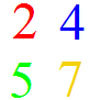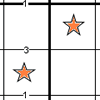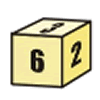Search by Topic

Resources tagged with Addition & subtraction similar to A One in Seven Chance:

Filter by: Content type:
Age range:
Challenge level:

There are 62 resultsChameleons

Age 11 to 14 Challenge Level:

Whenever two chameleons of different colours meet they change colour to the third colour. Describe the shortest sequence of meetings in which all the chameleons change to green if you start with 12. . . .Age 11 to 14 Challenge Level:

If you take a three by three square on a 1-10 addition square and multiply the diagonally opposite numbers together, what is the difference between these products. Why?Pyramids

Age 11 to 14 Challenge Level:

What are the missing numbers in the pyramids?Digit Sum

Age 11 to 14 Challenge Level:

What is the sum of all the digits in all the integers from one to one million?Always the Same

Age 11 to 14 Challenge Level:

Arrange the numbers 1 to 16 into a 4 by 4 array. Choose a number. Cross out the numbers on the same row and column. Repeat this process. Add up you four numbers. Why do they always add up to 34?Like Powers

Age 11 to 14 Challenge Level:

Investigate $1^n + 19^n + 20^n + 51^n + 57^n + 80^n + 82^n$ and $2^n + 12^n + 31^n + 40^n + 69^n + 71^n + 85^n$ for different values of n.Subtraction Surprise

Age 7 to 14 Challenge Level:

Try out some calculations. Are you surprised by the results?Got it for Two

Age 7 to 14 Challenge Level:

Got It game for an adult and child. How can you play so that you know you will always win?Magic Squares

Age 14 to 18

An account of some magic squares and their properties and and how to construct them for yourself.Largest Number

Age 11 to 14 Challenge Level:

What is the largest number you can make using the three digits 2, 3 and 4 in any way you like, using any operations you like? You can only use each digit once.Tis Unique

Age 11 to 14 Challenge Level:

This addition sum uses all ten digits 0, 1, 2...9 exactly once. Find the sum and show that the one you give is the only possibility.Aba

Age 11 to 14 Challenge Level:

In the following sum the letters A, B, C, D, E and F stand for six distinct digits. Find all the ways of replacing the letters with digits so that the arithmetic is correct.Eleven

Age 11 to 14 Challenge Level:

Replace each letter with a digit to make this addition correct.Top-heavy Pyramids

Age 11 to 14 Challenge Level:

Use the numbers in the box below to make the base of a top-heavy pyramid whose top number is 200.Have You Got It?

Age 11 to 14 Challenge Level:

Can you explain the strategy for winning this game with any target?Consecutive Negative Numbers

Age 11 to 14 Challenge Level:

Do you notice anything about the solutions when you add and/or subtract consecutive negative numbers?Calendar Capers

Age 11 to 14 Challenge Level:

Choose any three by three square of dates on a calendar page...Making Sense of Positives and Negatives

Age 11 to 14

This article suggests some ways of making sense of calculations involving positive and negative numbers.Postage

Age 14 to 16 Challenge Level:

The country Sixtania prints postage stamps with only three values 6 lucres, 10 lucres and 15 lucres (where the currency is in lucres).Which values cannot be made up with combinations of these postage. . . .Countdown

Age 7 to 14 Challenge Level:

Here is a chance to play a version of the classic Countdown Game.Crossed Ends

Age 11 to 14 Challenge Level:

Crosses can be drawn on number grids of various sizes. What do you notice when you add opposite ends?Number Pyramids

Age 11 to 14 Challenge Level:

Try entering different sets of numbers in the number pyramids. How does the total at the top change?And So on and So On

Age 11 to 14 Challenge Level:

If you wrote all the possible four digit numbers made by using each of the digits 2, 4, 5, 7 once, what would they add up to?Cunning Card Trick

Age 11 to 14 Challenge Level:

Delight your friends with this cunning trick! Can you explain how it works?Jugs of Wine

Age 11 to 14 Challenge Level:

You have four jugs of 9, 7, 4 and 2 litres capacity. The 9 litre jug is full of wine, the others are empty. Can you divide the wine into three equal quantities?Arrange the Digits

Age 11 to 14 Challenge Level:

Can you arrange the digits 1,2,3,4,5,6,7,8,9 into three 3-digit numbers such that their total is close to 1500?Magic Squares for Special Occasions

Age 11 to 16

This article explains how to make your own magic square to mark a special occasion with the special date of your choice on the top line.Constellation Sudoku

Age 14 to 18 Challenge Level:

Special clue numbers related to the difference between numbers in two adjacent cells and values of the stars in the "constellation" make this a doubly interesting problem.Clocked

Age 11 to 14 Challenge Level:

Is it possible to rearrange the numbers 1,2......12 around a clock face in such a way that every two numbers in adjacent positions differ by any of 3, 4 or 5 hours?Card Trick 2

Age 11 to 14 Challenge Level:

Can you explain how this card trick works?3388

Age 11 to 14 Challenge Level:

Using some or all of the operations of addition, subtraction, multiplication and division and using the digits 3, 3, 8 and 8 each once and only once make an expression equal to 24.As Easy as 1,2,3

Age 11 to 14 Challenge Level:

When I type a sequence of letters my calculator gives the product of all the numbers in the corresponding memories. What numbers should I store so that when I type 'ONE' it returns 1, and when I type. . . .Alphabet Soup

Age 11 to 14 Challenge Level:

This challenge is to make up YOUR OWN alphanumeric. Each letter represents a digit and where the same letter appears more than once it must represent the same digit each time.Kids

Age 11 to 14 Challenge Level:

Find the numbers in this sumThe Patent Solution

Age 11 to 14 Challenge Level:

A combination mechanism for a safe comprises thirty-two tumblers numbered from one to thirty-two in such a way that the numbers in each wheel total 132... Could you open the safe?Adding and Subtracting Positive and Negative Numbers

Age 11 to 14

How can we help students make sense of addition and subtraction of negative numbers?An Introduction to Magic Squares

Age 7 to 16Cubes Within Cubes

Age 7 to 14 Challenge Level:

We start with one yellow cube and build around it to make a 3x3x3 cube with red cubes. Then we build around that red cube with blue cubes and so on. How many cubes of each colour have we used?Cayley

Age 11 to 14 Challenge Level:

The letters in the following addition sum represent the digits 1 ... 9. If A=3 and D=2, what number is represented by "CAYLEY"?Got it Article

Age 7 to 14

This article gives you a few ideas for understanding the Got It! game and how you might find a winning strategy.Pole Star Sudoku 2

Age 11 to 16 Challenge Level:

This Sudoku, based on differences. Using the one clue number can you find the solution?Football Sum

Age 11 to 14 Challenge Level:

Find the values of the nine letters in the sum: FOOT + BALL = GAMEScore

Age 11 to 14 Challenge Level:

There are exactly 3 ways to add 4 odd numbers to get 10. Find all the ways of adding 8 odd numbers to get 20. To be sure of getting all the solutions you will need to be systematic. What about. . . .First Connect Three for Two

Age 7 to 14 Challenge Level:

First Connect Three game for an adult and child. Use the dice numbers and either addition or subtraction to get three numbers in a straight line.Nice or Nasty for Two

Age 7 to 14 Challenge Level:

Some Games That May Be Nice or Nasty for an adult and child. Use your knowledge of place value to beat your opponent.More Plant Spaces

Age 7 to 14 Challenge Level:

This challenging activity involves finding different ways to distribute fifteen items among four sets, when the sets must include three, four, five and six items.More Children and Plants

Age 7 to 14 Challenge Level:

This challenge extends the Plants investigation so now four or more children are involved.Twenty20

Age 7 to 16 Challenge Level:

Fancy a game of cricket? Here is a mathematical version you can play indoors without breaking any windows.2010: A Year of Investigations

Age 5 to 14

This article for teachers suggests ideas for activities built around 10 and 2010.Weights

Age 11 to 14 Challenge Level:

Different combinations of the weights available allow you to make different totals. Which totals can you make?